# Chapter 12 Income Taxes and Capital Budgeting Oleh

• Slides: 28Chapter 12 Income Taxes and Capital Budgeting Oleh Bambang KesitLearning Objective 1 Compute the after-tax net present values of projects.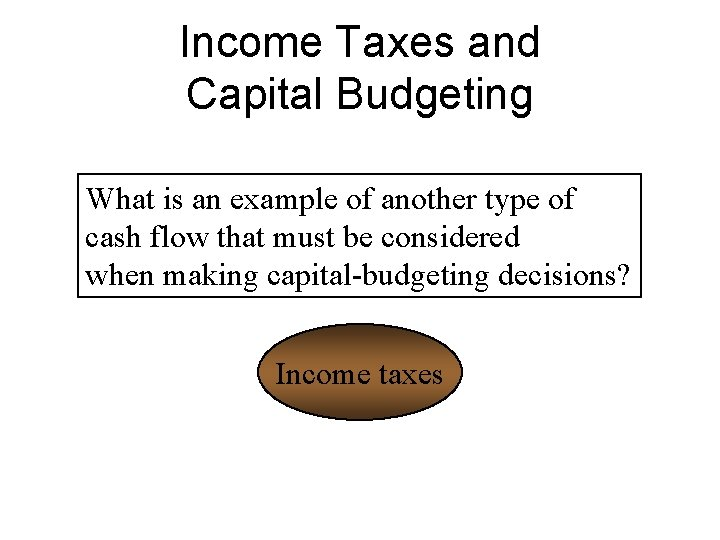Income Taxes and Capital Budgeting What is an example of another type of cash flow that must be considered when making capital-budgeting decisions? Income taxesMarginal Income Tax Rate • In capital budgeting, the relevant tax rate is the marginal income tax rate. • This is the tax rate paid on additional amounts of pretax income.Effects of Depreciation Deductions • For tax purposes, accelerated depreciation is generally allowed. • The focus is on the tax reporting rules, not those for public financial reporting. • The number of years over which an asset is depreciated for tax purposes is called the recovery period.Depreciation Deductions for Capital Budgeting • Depreciating a fixed asset creates future tax deductions. • The present value of this deduction depends directly on its specific yearly effects on future income tax payments.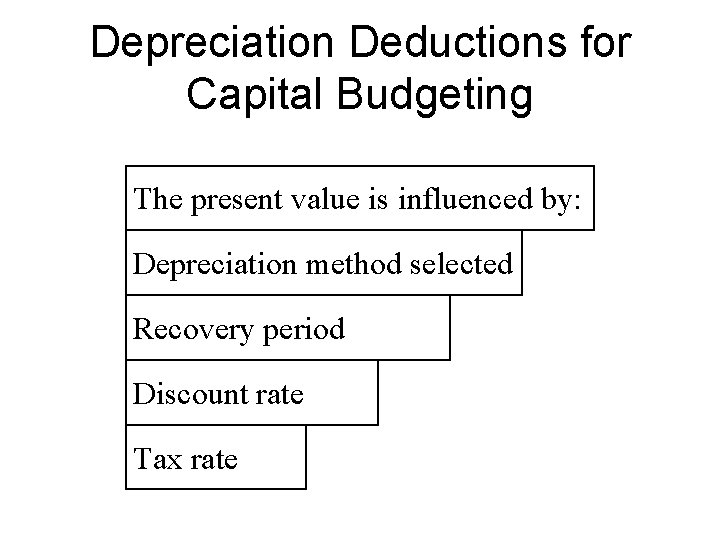Depreciation Deductions for Capital Budgeting The present value is influenced by: Depreciation method selected Recovery period Discount rate Tax rate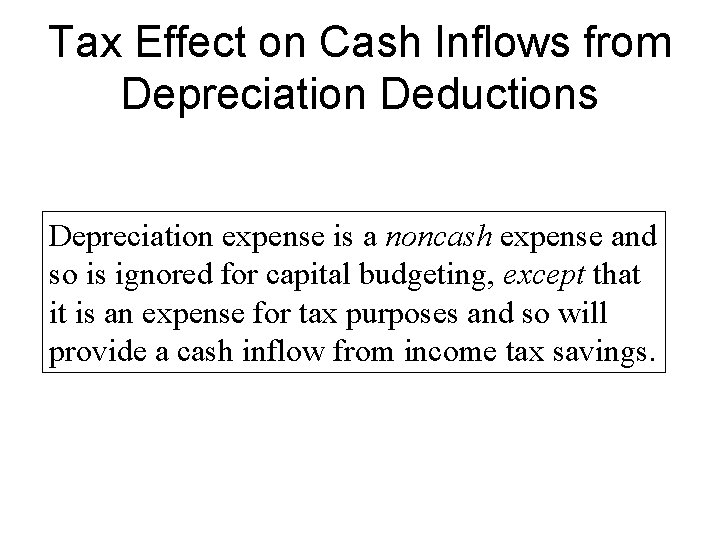Tax Effect on Cash Inflows from Depreciation Deductions Depreciation expense is a noncash expense and so is ignored for capital budgeting, except that it is an expense for tax purposes and so will provide a cash inflow from income tax savings.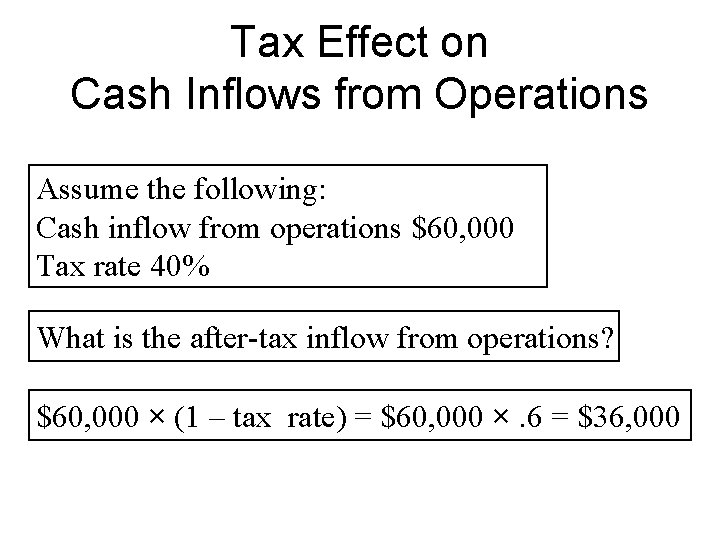Tax Effect on Cash Inflows from Operations Assume the following: Cash inflow from operations \$60, 000 Tax rate 40% What is the after-tax inflow from operations? \$60, 000 × (1 – tax rate) = \$60, 000 ×. 6 = \$36, 000Modified Accelerated Cost Recovery System • Under U. S. income tax laws, most assets purchased since 1987 are depreciated using the Modified Accelerated Cost Recovery System (MACRS). • This system specifies a recovery period an accelerated depreciation schedule for all types of assets.Learning Objective 2 Explain the after-tax effect on cash of disposing of assets.Gains or Losses on Disposal Suppose a piece equipment purchased for \$125, 000 is sold at the end of year 3 after taking three years of straight-line depreciation. What is the book value? \$125, 000 – (3 × \$25, 000) = \$50, 000Gains or Losses on Disposal • If it is sold for book value, there is no gain or loss and so there is no tax effect. • If it is sold for more than \$50, 000, there is a gain and an additional tax payment. • If it is sold for less than \$50, 000, there is a loss and a tax savings. TAX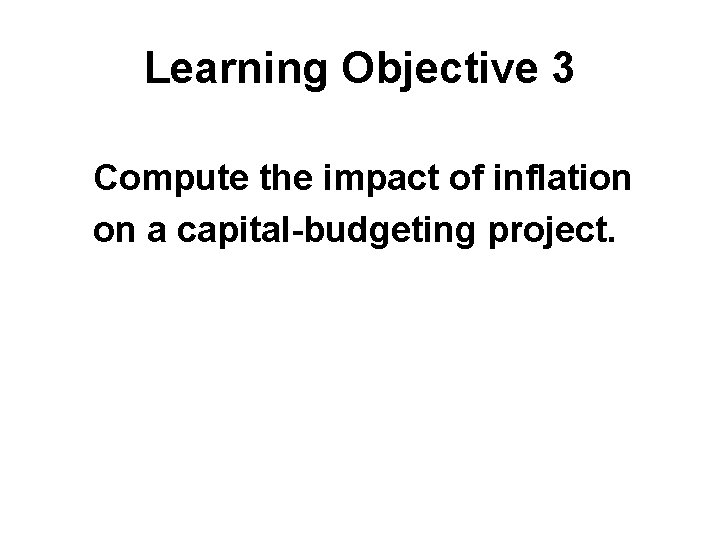Learning Objective 3 Compute the impact of inflation on a capital-budgeting project.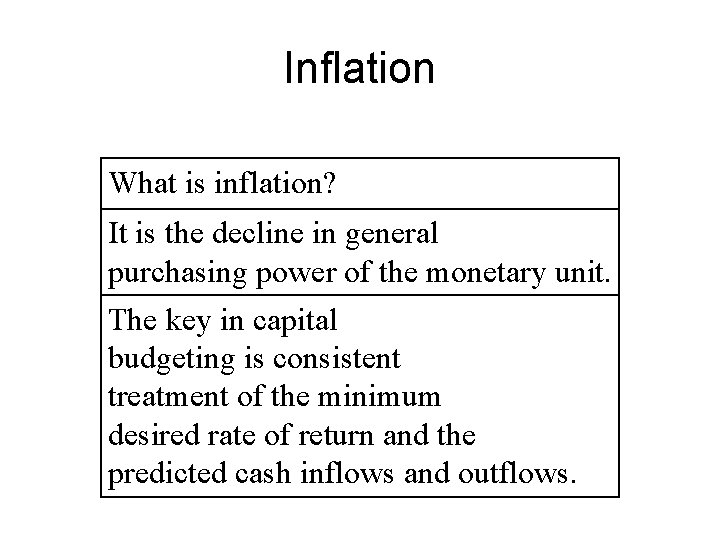Inflation What is inflation? It is the decline in general purchasing power of the monetary unit. The key in capital budgeting is consistent treatment of the minimum desired rate of return and the predicted cash inflows and outflows.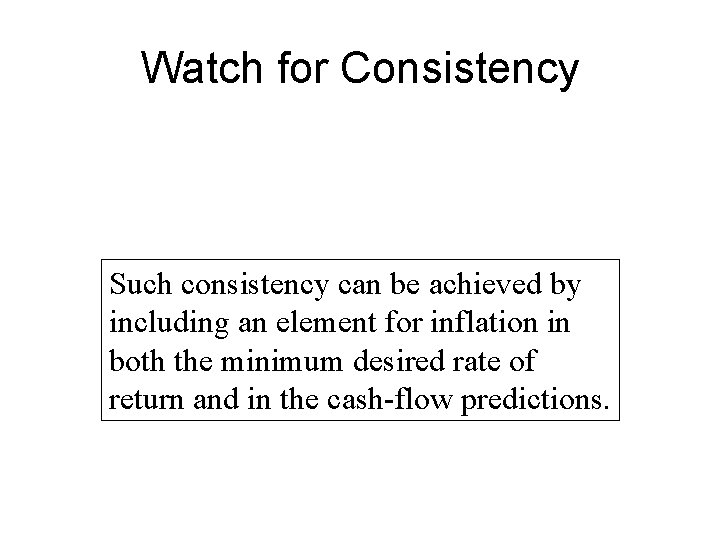Watch for Consistency Such consistency can be achieved by including an element for inflation in both the minimum desired rate of return and in the cash-flow predictions.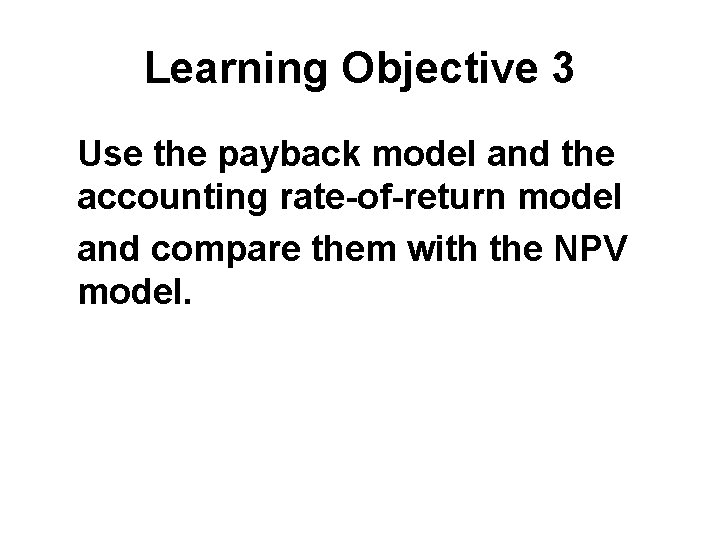Learning Objective 3 Use the payback model and the accounting rate-of-return model and compare them with the NPV model.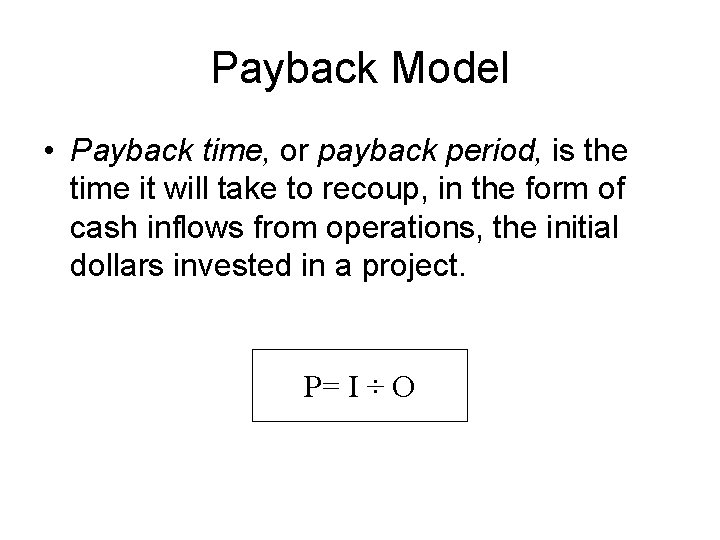Payback Model • Payback time, or payback period, is the time it will take to recoup, in the form of cash inflows from operations, the initial dollars invested in a project. P= I ÷ O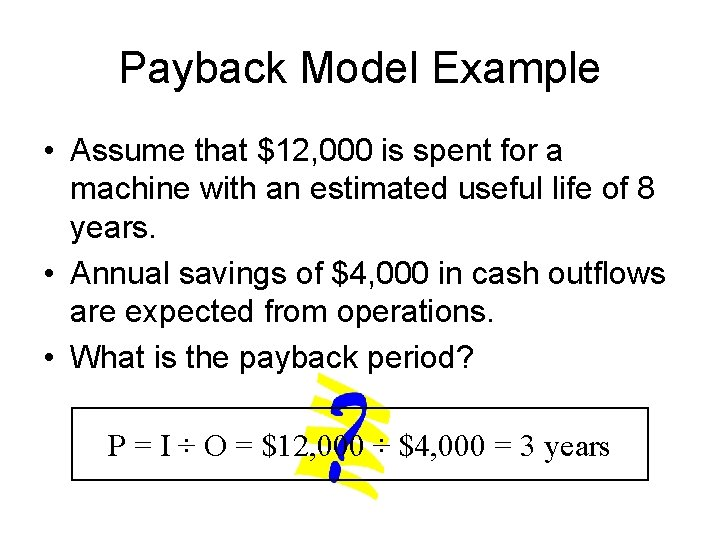Payback Model Example • Assume that \$12, 000 is spent for a machine with an estimated useful life of 8 years. • Annual savings of \$4, 000 in cash outflows are expected from operations. • What is the payback period? P = I ÷ O = \$12, 000 ÷ \$4, 000 = 3 years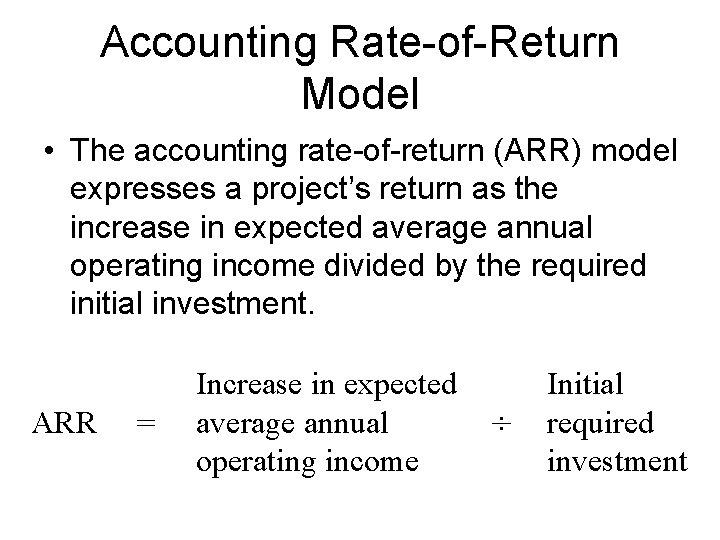Accounting Rate-of-Return Model • The accounting rate-of-return (ARR) model expresses a project’s return as the increase in expected average annual operating income divided by the required initial investment. ARR = Increase in expected average annual operating income ÷ Initial required investment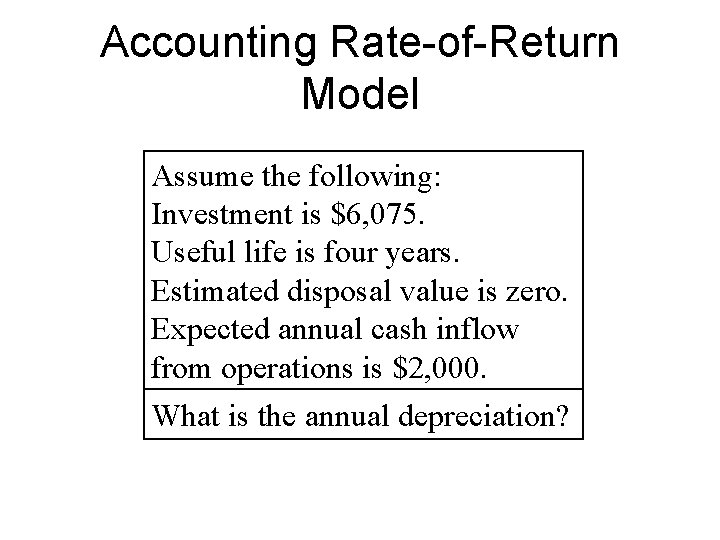Accounting Rate-of-Return Model Assume the following: Investment is \$6, 075. Useful life is four years. Estimated disposal value is zero. Expected annual cash inflow from operations is \$2, 000. What is the annual depreciation?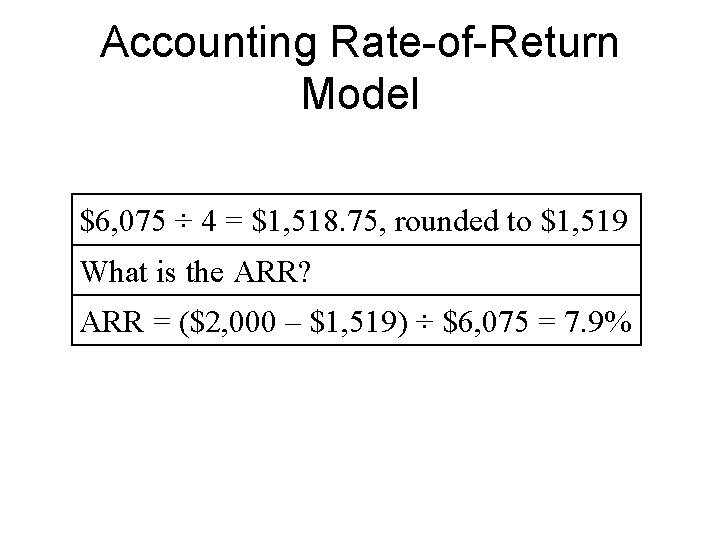Accounting Rate-of-Return Model \$6, 075 ÷ 4 = \$1, 518. 75, rounded to \$1, 519 What is the ARR? ARR = (\$2, 000 – \$1, 519) ÷ \$6, 075 = 7. 9%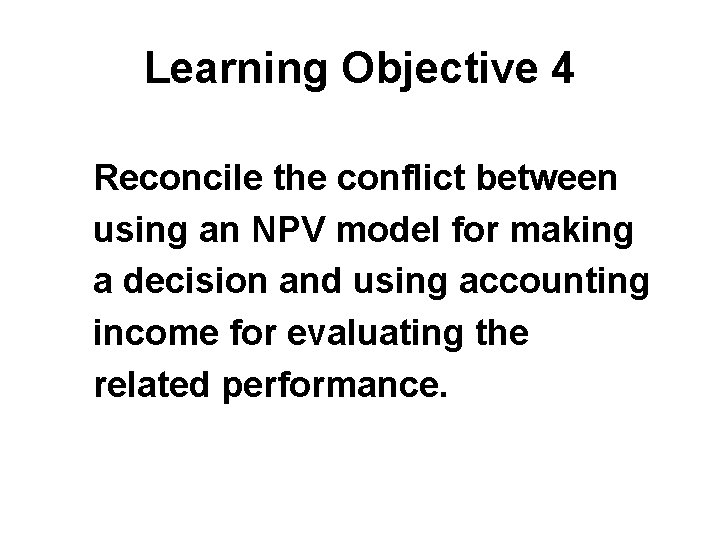Learning Objective 4 Reconcile the conflict between using an NPV model for making a decision and using accounting income for evaluating the related performance.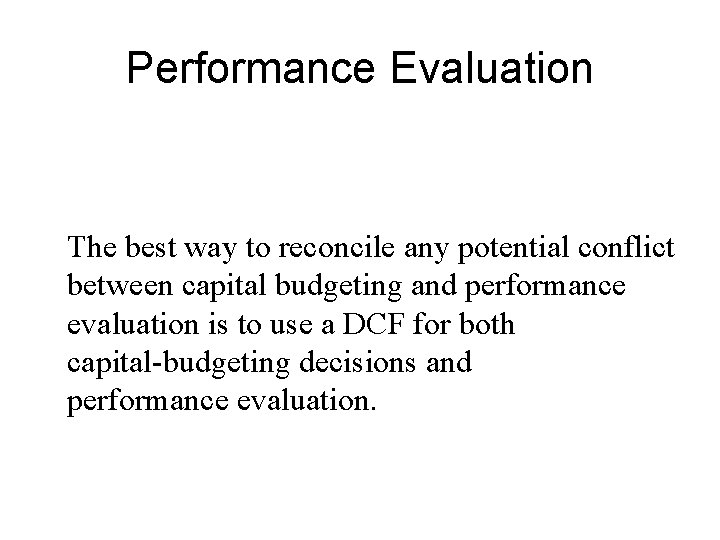Performance Evaluation The best way to reconcile any potential conflict between capital budgeting and performance evaluation is to use a DCF for both capital-budgeting decisions and performance evaluation.Post Audit • A recent survey showed that most large companies conduct a follow-up evaluation of at least some capital-budgeting decisions, often called a post audit. • The post audit focuses on actual versus predicted cash flows.Learning Objective 5 Understand how companies make long-term capital investment decisions and how such decisions can affect the companies’ financial results for years to come.Long-term Capital Investments… are critical to a company’s financial success. Using a discounted cash-flow method helps managers make optimal capital budgeting decisions.End of Chapter